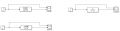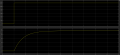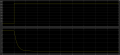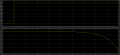# Transfer function response for step input

#### Papabravo

Joined Feb 24, 2006
20,146
Do you have any information on the order of the transfer functions? My choices would be 1st order or overdamped 2nd order. I see no information in the problem statement that would give a clue. Is there more information that you're not showing us?

#### crutschow

Joined Mar 14, 2008
32,062
Looks like a low-pass filter and an inverted low-pass filter.

Your graphs show PWM on the vertical axis but I see no info on that.
Are those input signals PWM?

#### 28sya

Joined Jun 13, 2020
20
Do you have any information on the order of the transfer functions? My choices would be 1st order or overdamped 2nd order. I see no information in the problem statement that would give a clue. Is there more information that you're not showing us?
I need to design a transfer function for a fixed wing UAV using the 1st order which give response based on step input. The input is PWM (thrust, drag) and the output response is airspeed. There are four cases, which are

Case 1 : PWM for thrust increase, airspeed increase
Case 2 : PWM for thrust decrease, airspeed decrease
Case 3 : PWM for drag increase, airspeed decrease
Case 4 : PWM for drag decreaase, airspeed increase

#### 28sya

Joined Jun 13, 2020
20
Looks like a low-pass filter and an inverted low-pass filter.

Your graphs show PWM on the vertical axis but I see no info on that.
Are those input signals PWM?
Yes, the input signals is PWM. How can I create a transfer function for low pass filter and inverted low pass filter?
I already did the transfer function but i couldn't get the response that i want. The transfer function that i need to design based on these cases

Case 1 : PWM for thrust increase, airspeed increase
Case 2 : PWM for thrust decrease, airspeed decrease
Case 3 : PWM for drag increase, airspeed decrease
Case 4 : PWM for drag decreaase, airspeed increase

the transfer function that i try to simulate in matlabStep input response for thrust up.Step input response for SpoilerUpStep input response for ThrustDown#### Papabravo

Joined Feb 24, 2006
20,146
Pretty nice. So they are all 1st order transfer functions. What was it that led you to those solutions?

#### 28sya

Joined Jun 13, 2020
20
Pretty nice. So they are all 1st order transfer functions. What was it that led you to those solutions?
Since I need to model the transfer function to get the response, thus i just assume input, output and sampling time (Ts). for example for the ThrustUp is
Input : 1200PWM
Output : 24m/s
Ts : 3s

for SpoilerUp, i almost got the solution but the amplitude should be in positive, so i think maybe i have the problem with the transfer function.

for ThrustDown, i don't know to find the correct transfer function

#### Papabravo

Joined Feb 24, 2006
20,146
Since I need to model the transfer function to get the response, thus i just assume input, output and sampling time (Ts). for example for the ThrustUp is
Input : 1200PWM
Output : 24m/s
Ts : 3s

for SpoilerUp, i almost got the solution but the amplitude should be in positive, so i think maybe i have the problem with the transfer function.

for ThrustDown, i don't know to find the correct transfer function
It shouldn't be too hard to test all possible 1st order transfer functions. If that doesn't work you might try a damped 2nd order function for the denominator with a compensating zero in the numerator. You could also take the root locus approach. If the system is going to be first order all the poles will be on the negative real axis.
With second order systems, the poles are conjugate pairs, and the zero is near the origin.

#### 28sya

Joined Jun 13, 2020
20
It shouldn't be too hard to test all possible 1st order transfer functions. If that doesn't work you might try a damped 2nd order function for the denominator with a compensating zero in the numerator. You could also take the root locus approach. If the system is going to be first order all the poles will be on the negative real axis.
With second order systems, the poles are conjugate pairs, and the zero is near the origin.
what are the first order system for step response?

#### Papabravo

Joined Feb 24, 2006
20,146
I think this is it for 1st order responses

#### 28sya

Joined Jun 13, 2020
20
I think this is it for 1st order responses
Whoaa... Thank you for your effort, i'm really appreciate it, but can you please write the transfer function equation that you used because i never used this kind of software. Is the green colour is the input? By the way, what software did you used?

#### Papabravo

Joined Feb 24, 2006
20,146
The transfer functions are on the schematic where the expression is Laplace = <Transfer Function> , and that is where I wrote them
The graphs are labeled V(out1) or Voltage at node OUT1
and V(out2) or voltage at node OUT2

It is a simulator (free download) that can simulate the response of arbitrary transfer functions expressed as their Lapalce transform.
It is called LTspice and it is a free download from the Analog Devices Inc. website. Many people on this site (AAC) use it for divers purposes

The E1 and E2 components are Voltage Dependent Voltage Sources

V1 is a positive step generator (0 --> 1) and V2 is a negative step generator (1 --> 0)

You can do either a transient analysis or Magnitude and Phase (Bode) plot

Last edited:

#### crutschow

Joined Mar 14, 2008
32,062
You can do either a transient analysis or Magnitude and Phase (Bode) plot
You can use the transient analysis if you want to see the response with an applied PWM signal as well as a step.

#### Papabravo

Joined Feb 24, 2006
20,146
You can use the transient analysis if you want to see the response with an applied PWM signal as well as a step.
I had not thought of that but you are right.

#### 28sya

Joined Jun 13, 2020
20
do
The transfer functions are on the schematic where the expression is Laplace = <Transfer Function> , and that is where I wrote them
The graphs are labeled V(out1) or Voltage at node OUT1
and V(out2) or voltage at node OUT2

It is a simulator (free download) that can simulate the response of arbitrary transfer functions expressed as their Lapalce transform.
It is called LTspice and it is a free download from the Analog Devices Inc. website. Many people on this site (AAC) use it for divers purposes

The E1 and E2 components are Voltage Dependent Voltage Sources

V1 is a positive step generator (0 --> 1) and V2 is a negative step generator (1 --> 0)

You can do either a transient analysis or Magnitude and Phase (Bode) plot
Do you know how to get this response if using transfer function block using simulink in matlab software?

#### Papabravo

Joined Feb 24, 2006
20,146
do

Do you know how to get this response if using transfer function block using simulink in matlab software?
Nope. I haven't been able to afford that package since I was a graduate student for the 2nd time in the early part of this century. They let us have it for the low-low price of \$99.00+tax -- of course.

#### MrAl

Joined Jun 17, 2014
10,087
View attachment 240762
How can i get these four transfer functions response based on the step input? What are the transfer function to get the output response?
Since a first order low pass filter has already been suggested, the general form of the transfer function is:
F(s)=a/(s+b)

and if you transform that into the time domain you get an exponential response:
f(t)=(a/b)*(1-e^(-b*t))

Looking at the first response which goes high and then low again...
Since your response looks perfectly flat for a time that would imply that the response is pinned at the level a/b for some time which implies that 'b' is large enough to get the response to go to the max well within the time of the length of the drive pulse, and that 'a/b' is the highest point on the output response just before the pulse goes back low again.
These observations suggest that there are two points on the drawn response curve where you can fit the time response to the curve:
1. Using a/b as the max amplitude you can set a/b=max, so the first equation is a/b=max.
2. Using a point on the rising part of the curve equal to 1-1/e (about 0.632) times a/b you can solve for 'b'.
3. Knowing 'b' you can insert that in the first equation and solve for 'a'. You now have both a and b which means you can write both the frequency domain response and the time domain responses.

That is one way to do it which is more or less exact because it uses a curve fitting technique. It is exact for a first order curve as described.